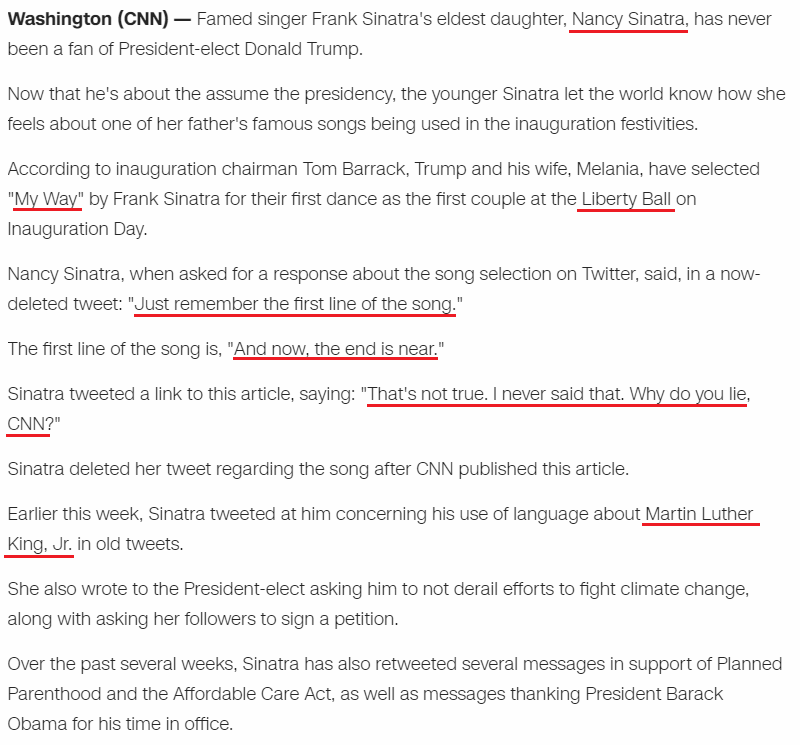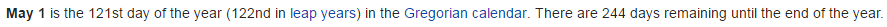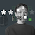CipherJan 21, 2017

Assassination Coding for Trump once again

This is second post I am making on Trump assassination, it's truly unbelievable how they come up with these crazy connections

This is the link from the first post from November https://nasanumerology.blogspot.rs/2016/11/possible-assassination-date-for-trump.html

Trump has over the top Saturnian coding surrounding him, and this perfectly follows the script as alwaysIt's incredible how much they gave us in such little post...
Nancy Sinatra = 5+1+5+3+7+10+9+5+1+2+9+1 = 58 (S Exception)
1/20/2017 = 1+20+20+17 = 58 (Trump won 58th quadrennial presidential election)

Fifty-eight = 115 (Trump ran for presidency for 511 days.. 511 converted to Octal numbering system is 777, he's getting into office at 70 years, 7 months, 7 days of age, also if we take 511 and add it with all possible combinations of these numbers we will end up with 777)
511+151+115 = 777 (Jesus Christ = 151 (36th prime) - 36th triangular number is 666, Magic square of the Sun (Son) sums to 666)
Also he's entering office with 115th congress
Trump was born on 6/14
Fourteenth of June = 6+6+3+9+2+5+5+5+2+8+6+6+1+3+5+5 = 77 (Reduced)
77 converted to Octal is 115

Also they say this
"Nancy Sinatra, has never been a fan of president-elect Donald Trump"

Excluding the name this has the same gematria like the first line of the song that was chosen for their first dance at "Liberty ball"

193 is 44th prime number
Kill = 11+9+12+12 = 44 (Ordinal)
Liberty ball = 12+9+2+5+18+20+25+2+1+12+12 = 118 (Ordinal)
Death = 4+5+1+100+8 = 118 (Jewish)

Trump came out on cover of Time magazine 44 days before Inauguration, May first (the date I predicted for his death) is also 44 days before his birthday
As soon as I started decoding it became very obvious why this song was chosen
The song and the album are called "My Way"
My Way = 13+25+23+1+25 = 87 (Ordinal)
eight seven = 5+9+7+8+100+90+5+700+5+40 = 969 (Jewish)
My Way = 39+25+49+1+25 = 139 (Francis Bacon)

Lenght is 4:35 ... 435+543 - 969 - Speed of Saturn
Length of  the song is 275 seconds (275th prime is 1777 which is interesting because of all 777 Trump coding connecting to Saturn everywhere)

Everything from this picture has very relevant gematria
The writers have interesting gematria
Paul Anka and Claude Francois = 70+1+300+30+1+50+20+1+1+50+4+3+30+1+300+4+5+6+90+1+50+3+60+9+100 = 1190 (English)
Donald = 4+50+40+1+20+4 = 119 (Jewish)
Claude Francois = 3+3+1+3+4+5+6+9+1+5+3+6+9+1 = 59 (Reduced)
17th prime number is 59

Paul Anka = 60+1+200+20+1+40+10+1 = 333 (Jewish)
Paul Anka = 7+1+3+3+1+5+2+1 = 23 (Reduced)
Donald = 4+6+5+1+3+4 = 23 (Reduced)

Paul Anka and Claude Francois = 16+1+21+12+1+14+11+1+1+14+4+3+12+1+21+4+5+6+18+1+14+3+15+9+19 = 227 (Ordinal)
Twenty two divided by seven = 20+23+5+14+20+25+20+23+15+4+9+22+9+4+5+4+2+25+19+5+22+5+14 = 314 (Ordinal)
Seven hundred seventy seven = 19+5+22+5+14+8+21+14+4+18+5+4+19+5+22+5+14+20+25+19+5+22+5+14 = 314 (Ordinal)
Weapons of Mass Destruction = 23+5+1+16+15+14+19+15+6+13+1+19+19+4+5+19+20+18+21+3+20+9+15+14 = 314 (Ordinal)

Label and producer have the same name gematria

45 is the sum of Magic square of Saturn
Ninety = 5+9+5+5+2+7 = 33 (Reduced)
But there is something very interesting with the producer of this song
Duke Ambassadors = 4+3+2+5+1+4+2+1+1+1+1+4+6+9+1 = 45 (Reduced)
Duke Ambassadors = 4+21+11+5+1+13+2+1+19+19+1+4+15+18+19 = 153 (Ordinal)

17th triangular number is 153, reflection of 26th triangular number 351, God = 17/26
December 17th is 351st day on which Romans celebrated their holiday Saturnalia
Also he was born in 'Scranton'
Scranton = 90+3+80+1+40+100+50+40 = 404 (Jewish)
Cancer = 3+1+14+3+5+18 = 44 (Ordinal)

He was born on 3/22 - 322 is number of Skull and Bones, also the reflection is very interesting, Masonic = 223 (J), The Synagogue of Satan = 223 (O)

His birth date numerology syncs perfectly with birthday of Frank Sintatra and both connect to date numerology of Inauguration day
3/22/1914 - 3+22+19+14 = 58 (Trump is coded with 58 all over)
3/22/1914 - 3+22+1+9+1+4 = 40
3/22/1914 - 3+2+2+1+9+1+4 = 22
3/22/14 = 39 (Saturn = 93, and he is worshiped by the Jews that go by 39 books of Old Testament, Trump's father died at the age of 93)
12/12/1915 - 12+12+19+15 = 58 (Freemasonry = 58, Secret Society = 58, Zodiac = 58, Calendar = 58)
12/12/1915 - 12+12+1+9+1+5 = 40
12/12/1915 - 1+2+1+2+1+9+1+5 = 22
12/12/15 = 12+12+15 = 39 (in this post they are talking to Inauguration chairman "Tom Barrack" = 39)

Frank Albert Sinatra = 6+9+1+5+2+1+3+2+5+9+2+1+9+5+1+2+9+1 = 73 (Reduced)
21st prime number is 73
Francis albert Sinatra = 6+18+1+14+3+9+19+1+12+2+5+18+20+19+9+14+1+20+18+1 = 210 (Ordinal)
Frank Albert Sinatra = 6+80+1+40+10+1+20+2+5+80+100+90+9+40+1+100+80+1 = 666 (Jewish)

The song 'My Way'  was recorded on 12/30/1968 - 12/30 reduced .. 1+2/3+0 .. 3/3
Conspiracy = 123
From 48th anniversary of release of this song to my predicted date for Trump is 123 days
Donald Trump = 4+6+5+1+3+4+2+9+3+4+7 = 48 (Reduced)
12/30 .. 12+30 = 42 (4 months, 2 days, 42 is one of those special numbers that even connected to Saturn through Magic Cube of Saturn and 42nd triangular number which is 903

12/30/1968 - 12+30+1+9+6+8 = 66 (Sonny Burke died at the age of 66, this one song seems to be connecting 2 people heavily already, maybe even the 3rd very soon)
Thirty-three = 2+8+9+9+2+7+2+8+9+5+5 = 66 (Reduced)
12/30/68 = 110 (Sonny Burke died in Santa Monica = 110)
President = 16+18+5+19+9+4+5+14+20 = 110 (Ordinal)
Also Frank Sinatra has interesting name gematria connecting to my prediction date for Trump
Frank Sinatra = 6+9+1+5+2+1+9+5+1+2+9+1 = 51 (Reduced)
First of May = 6+9+9+1+2+6+6+4+1+7 = 51 (Reduced)
Conspiracy = 3+6+5+1+7+9+9+1+3+7 = 51 (Reduced)
Getting back to CNN's post
They gave us something really interesting in quotation marks
"Just remember the first line of the song."
"And now, the end is near."
"That's not true. I never said that. Why do you lie, CNN?"
Just remember the first line of the song = 1+3+10+2+9+5+4+5+4+2+5+9+2+8+5+6+9+9+10+2+3+9+5+5+6+6+2+8+5+10+6+5+7 = 187 (S Exception
That's not true. I never said that. Why do you lie, CNN? = 2+8+1+2+1+5+6+2+2+9+3+5+9+5+5+4+5+9+1+1+9+4+2+8+1+2+5+8+7+4+6+7+6+3+3+9+5+3+5+5 = 187 (Reduced)
187 is the Homicide code
One hundred eighty seven = 2+1+5+6+6+1+4+5+5+4+5+5+7+6+7+2+6+5+5+5+1 = 93 (Septenary)
Homicide Code = 8+15+13+9+3+9+4+5+3+15+4+5 = 93 (Ordinal)

Homicide code = 8+6+4+9+3+9+4+5+3+6+4+5 = 66 (Reduced)
Thirty three = 2+8+9+9+2+7+2+8+9+5+5 = 66 (Reduced)
Also in the post they say that Nancy Sinatra was tweeting about Martin Luther King Jr. which is very interesting

Thirty-three = 2+8+9+9+2+7+2+8+9+5+5 = 66 (Reduced)
Michael King Jr = 4+9+3+8+1+5+3+2+9+5+7+1+9 = 66 (Reduced)

He was born on 1/15 (Saturn = 511 - Jewish)
1/15/29 = 45 (Today Trump became the 45th president of the USA)
Fifty-eight = 6+9+6+20+25+5+9+7+8+20 = 115 (Ordinal)
58 date numerology today, this year was 58th election, Trump's daughter is named Ivanka
Ivanka = 9+22+1+14+11+1 = 58 (Ordinal)

Martin Luther King Jr = 4+1+9+2+9+5+3+3+2+8+5+9+2+9+5+7+1+9 = 93 (Reduced)
Saturn = 19+1+20+21+18+14 = 93 (Ordinal)
Died at the age of 39 on April 4th (4/4)
Death = 24+30+6+120+48 = 228 (Sumerian)
If Trump died on May First 2017 it would be 244 days before 49th (7x7) anniversary of recording of the song "My Way"
Trump was born on 6/14
Fourteenth of June = 6+6+3+9+2+5+5+5+2+8 + 6+6 + 1+3+5+5 = 77 (Reduced)
7 months, 30 days - 73 (21st prime , Saturn = 21)Very interesting that they mention a man who was assassinated on top of all this coding...

Look out for 5/1/2017 or 5/11/2017... It's gonna be very interesting to see where they take all of this

1.1.